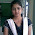Annoucement: New Java and Selenium batch is starting from 9th Jan 2020, Please enroll ASAP!

### How to find Armstrong Number

How to find Armstrong Number

Learn:
1. check the given number is Armstrong or not.
2. write different test cases for Armstrong Number

Code:

package Questions;

public class ArmstrongNumber {
//153
//1*1*1 = 1
//5*5*5 = 125
//3*3*3 = 27
//1+125+27 = 153
//407 == 4*4*4 + 0 + 7*7*7 = 407
//0
//1 == 1*1*1 = 1
//370, 371
public static void isArmstrongNumber(int num){
//153 ==  1*1*1    5*5*5      3*3*3
System.out.println("given number is "+ num );
int cube = 0;
int r;
int t;
t=num;
while(num>0){
r = num%10;
num = num/10;
cube = cube+(r*r*r);
}
if(t==cube){
System.out.println("this is an armstrong number");
}else{
System.out.println("this is not an armstrong number");
}
}

public static void main(String[] args) {
isArmstrongNumber(153);
isArmstrongNumber(370);
isArmstrongNumber(0);
isArmstrongNumber(1);
isArmstrongNumber(455);
}
}

1.2.